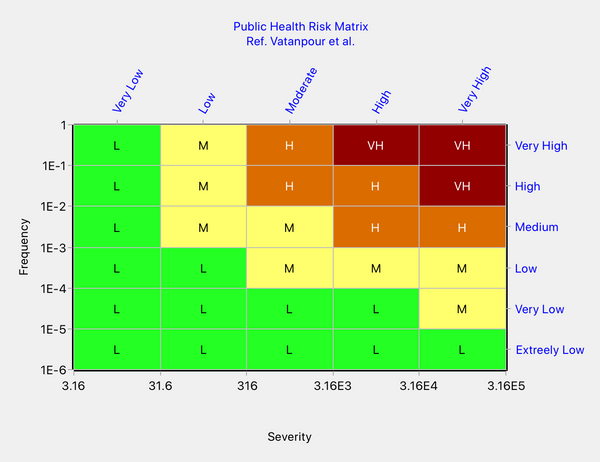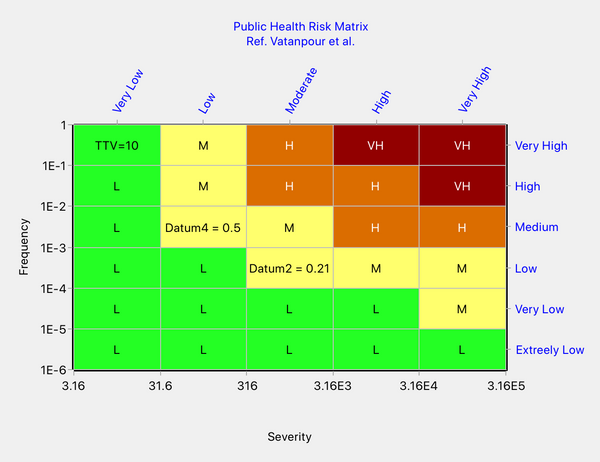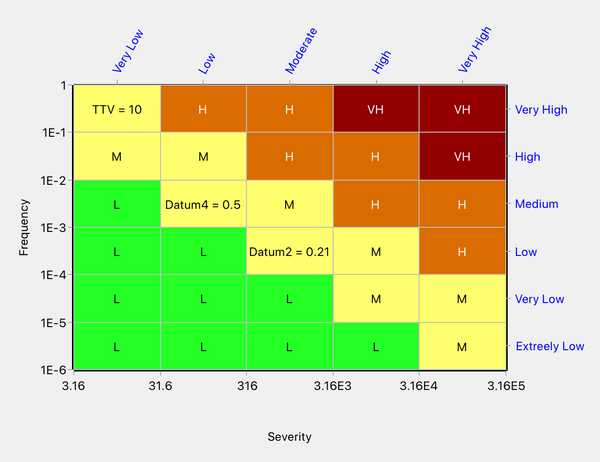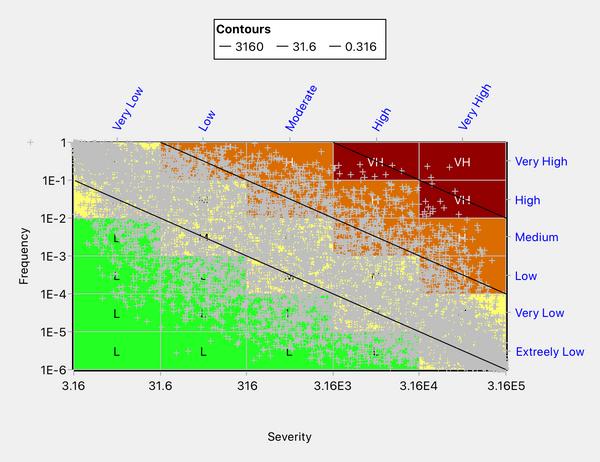# How Not to Design a Risk Matrix - A Public Health Risk Matrix

In a another post, we discussed a concern expressed by Cox (Ref. 1) that risk matrices may be "worse than useless" when probabilities and consequences are negatively correlated. We showed in that post that the concern only arises in highly contrived situations.

A paper by Vatanpour et al. of the University of Alberta (Ref. 2) is particularly interesting because it attempts to investigate Cox's concern by reference to real-world data from the public health field. The authors conclude that Cox's concern is valid, but we shall show in this post that their conclusion is illusory and due to use of a poorly designed risk matrix.

The risk matrix used by Vatanpour et al., redrawn with axes to scale, and displaying ratio scales as well as categories, is as follows:Figure 1: Risk matrix of Vatanpour et al.

The frequency scale is as stated by Vatanpour et al. The severity scale was not explicitly given but we noted that severity was scored as 10, 100, 1000, 1E4 and 1E5 for the five categories, respectively. Our tick marks were calculated to place the given severity scores approximately at the geometric mean of each severity category. For example, the geometric mean of 3.16 and 31.6 is approximately 10. The matrix classifies risks into four risk priority levels, ranging from Low to Very High.

Vatanpour et al. plotted the risks associated with a number of blood-borne infectious diseases on their matrix. The plotted points exhibited negative correlation, as would be expected (since more severe consequences tend to be less frequent). The Spearman correlation coefficient was -0.81. Due to a paucity of real-life data, they also included four risk points that were fictitious but chosen to fit with the general trend. The artificial data points were called Datum 1 through Datum 4.

The authors observed that Datum 2 (empirical risk = 0.21) and Datum 4 (empirical risk = 0.5) were rated as Medium risk whereas TT Virus (empirical risk = 10) was rated as Low risk - see Figure 2 below. This type of anomaly, where larger risks are ranked lower than smaller risks, is called rank reversal.Figure 2: The rank reversal anomaly observed by Vatanpour et al.

Vatanpour et al. attempt to explain the anomaly as follows:

"The generated data points 2 and 4 have estimated risk values of 0.21 and 0.50 and both are categorized in Figure 5 as medium risks. When compared with TT virus, which was categorized as a low risk in Figure 5, we find that it has an estimated (according to Equation (4)) risk of 10. This anomaly illustrates the concern posed by Cox, that the risk assessment matrix provides a risk categorization (color code) that is incorrect in relation to an empirical calculation of the risk . Although we had to resort to generating data from an empirical relationship derived from experiential frequency estimates, we have found that the theoretical concern of Cox can be demonstrated for hazard data derived from authentic experience."

It is surprising that the authors did not discuss the possible alternative explanation that the rank reversal error might be due to inadequate risk matrix design. It is also surprising that this possibility was not picked up in the journal's peer review process.

Consider the cells in which Datum 2 and Datum 4 are located. Points in either of these cells can have risks ranging from 0.0316 at the bottom left to 3.16 at the top right. Compare with the cell containing the data point relating to TT virus (TTV). The risk range for the cell containing the TTV data point is 0.316 to 31.6, i.e. risks in this cell are 10 to 100 times higher than in the cells containing Datum 2 and Datum 4. Therefore, if the yellow coloring is correct for the latter cells, then the cell containing the TTV data point cannot be green but must be at least yellow.

Thus, the authors have not validated the theoretical concern of Cox but instead have vividly demonstrated a common error in risk matrix construction, which is to fail to properly account for the axis scales when determining the matrix coloring pattern.

We will redesign the authors' risk matrix using Quick Risk Matrix. Unfortunately, the authors did not state the quantitative values defining the four risk zones. We will assume that the three iso-risk contours separating the four risk zones take the values 3160, 31.6 and 0.316. Thus, each risk threshold is a factor of 100 lower than the one above it. This gives a risk graph as shown below. Quick Risk Matrix is based on the concept that one develops a risk graph and then converts it to a discrete risk matrix. Several different algorithms are provided for making the conversion.Figure 3: Risk graph to serve as the basis for the redesign of the risk matrix

Since this risk matrix is for use in connection with the important safety issue of bloodborne disease, it is prudent to use the Round-Up algorithm, which colors each split cell according to the highest risk zone in it. The Round-Up algorithm ensures that any errors will be the safe side, i.e. the risk level will never be underestimated. With the Round-Up algorithm, we arrive at the following design:Figure 5: Redesign in Quick Risk Matrix based on the Round-Up algorithm

Note that our redesign has eliminated the rank reversal anomaly observed by Vatanpour et al. All three data points are now ranked the same, i.e. medium risk.

Our redesign has a coloring pattern substantially different to that of Vatanpour et al. This suggests that if we look hard enough at the design of Vatanpour et al., we should be able to detect some inconsistencies. This is indeed the case. For example, compare the cells in column 2, row 6, and column 3, row 5. Points in both these cells lie in the risk range 3.16 to 316. In the design of Vatanpour et al. (see Figure 1 or 2), these two cells have different colors. Since these cells have identical risk range, they should have the same color. In fact, with these axes, where the tick mark values increase by a factor of 10 as we move across the columns or rows, the risk range is identical for all cells lying on any diagonal line having a slope of -1. The color should, therefore, be the same for all cells lying on such a diagonal. Informed by this observation, we can see that the risk matrix design of Vatanpour et al. contains multiple incorrectly colored cells.

We can benchmark the accuracy of our redesign using the performance simulation tool in Quick Risk Matrix Premium. We assume that the frequency and severity have a log-uniform distribution with a Spearman correlation coefficient of -0.81 (the value given by Vatanpour et al.). We find that the Round-Up algorithm is predicted to achieve a mapping accuracy of 76% with all errors on the safe side. If we simulate for pairs of random points, the percentage error in ranking the two points of a pair is found to be 0.7% if the two points lie in different colored regions and 23% if they do not necessarily lie in different colored regions. (We explain in another post how all these benchmarks are calculated.)

Note that the benchmarks do not necessarily accurately predict real-world performance but are intended only for comparing different risk matrix designs.

Our final illustration shows a sample of points generated, with the above-described negative correlation, plotted on the risk matrix produced by the Round-Up algorithm. A substantial proportion of the generated points lie in cells split by iso-risk contours, which explains why the mapping accuracy is relatively low at 76%. Mapping errors are inevitable when using a risk matrix rather than a risk graph but, if we are concerned about them, we can use the round-up algorithm to ensure that errors are on the safe side.Figure 6: A sample of negatively correlated points used to benchmark the accuracy of the risk matrix

It is concluded that the risk matrix of Vatanpour et al. did not validate Cox's concern relating to negative correlations but instead demonstrated a common error in risk matrix design, namely not adequately considering the axis scales.

Ref. 1, Cox, L.A., What’s Wrong with Risk Matrices? Risk Analysis, Vol. 28, No. 2, 2008.

Ref. 2. Vatanpour S., Hrudey S.E., and Dinu, I., Can Public Health Risk Assessment Using Risk Matrices Be Misleading? Int. J. Environ. Res. Public Health 2015, 12, 9575-9588.